EDIT MAIN

Welcome to the 4th grade SMART Pages! Find information on what your child is learning, vocabulary, activities for home, and learning links!

 1st Quarter 2nd Quarter 3rd Quarter 4th Quarter Numbers and Operations in Base Ten Operations and Algebraic Thinking Operations and Algebraic Thinking Numbers and Operations in Fractions Numbers and Operations in Fractions Geometry Geometry Measurement and Data Whole Numbers, Place Value and Rounding in Computation Multiplication and Division of Whole Numbers Multiplication and Division of Whole Numbers Fraction Equivalents Fractions and Decimals Geometry Measurement Show what you know!
##### Numbers & Operations in Base Ten

 Understand multi-digit place value to 1,000,000. (4.NBT.1) Quarter 1, 2, 3, and 4 Read, write, and represent multi-digit whole numbers in standard and expanded form. (4.NBT.2) Quarter 1, 2, 3, and 4 Compare two multi-digit numbers using >, =, and <. (4.NBT.2) Quarter 1, 2, 3,and 4 Round multi-digit whole numbers. (4.NBT.3) Quarter 1, 2, 3, and 4 Fluently add and subtract multi-digit numbers. (4.NBT.4) Quarter 1, 2, 3, and 4 Multiply multi-digit numbers and explain the calculations by using equations, rectangular arrays, and/or area models. (4.NBT.5) Quarter 1, 2, 3, and 4 Divide multi-digit dividends with one-digit divisors, including interpreting the remainders. (4.NBT.6) Quarter 1, 2, 3, and 4
* Grade 4 students work with whole numbers less than or equal to 1,000,000.
Vocabulary
 Place Value: The value of a digit in a number Digit: A symbol used to show a number Estimate: An approximate calculation that is close to the exact number based on rounding Compare: Tell how a number is related to another number using >, <, =, or different Rounding: to find the nearest ten, hundred, or thousand (and so on). For example, 391 rounds up to 400 and 331 rounds up to 300. Expanded Form: Writing a number as the sum of the values in each digit Regrouping: To exchange amounts of equal value to rename a number

Activities At Home
• Make up numbers, roll numbers with dice, or find numbers (on labels) and compare them
• Find numbers and write them in expanded form
• Make numbers and tell which place value (and/or value) each digit represents
• Place large numbers on a number line
• Collect objects (i.e. Cheerios) and estimate how many
• Draw pictures and make models of numbers
• Practice addition and subtraction facts

 Ten Frames Compare Numbers Quick Math Equivalent Numbers Place Value Blocks Balanced Equations Place Value Creating Numbers Number Puzzles Interactive Number Line Expanded Form Deep Sea Duel Math Lines Base Ten Blocks Connect the Dots Multiples Division Drag Race Rounding Numbers Rounding to Tens Spaceships Rounding to Hundreds Sharks Compare Numbers Crossword Using Partial Method Multiplication Making Arrays Activity Factors Using Arrays
##### Operations & Algebraic Thinking

 Interpret multiplication as comparison, for example, 35 is 5 times as many as 7. (4.OA.1) Quarter 1, 2, 3, and 4 Multiply or divide to solve word problems (4.OA.2) Quarter 1,2,3, and 4 Solve multi-step word problems using the four operations. Problems will include interpreting a remainder and/or using equations with a letter standing for the unknown. (4.OA.3) Quarter 2 and 4 Find factor pairs for numbers to 100 and tell if numbers are prime or composite. (4.OA.4) Quarter 1, 2, 3, and 4 Understand Patterns with numbers or shapes. (4.OA.5) Quarter 2, and 4
Vocabulary
 Factor: A number that is multiplied by another (factor x factor = product) Regrouping: To exchange amounts of equal value to rename a number Expanded Form: a graph that shows the frequency of data along a number line Multiple: The product of a given whole number and another whole number Compare: Tell how a number is related to another number using >, <, =, or different Array: An arrangement of objects in rows and columns Identity Property: (Any number times 1 is that number Commutative Property: 5 x 7 is the same as 7 x 5 Associative Property: (2 x 3) x 4 = 2 x (3 x 4) Zero Property: Any number times 0 is 0 Product: The answer to a multiplication problem Remainder: The amount left over when a number cannot be divided equally Divisor: The number that divides the dividend Dividend: A number being divided Quotient: the result of a division Estimate: To find a number close to an exact amount

Activities At Home
• Draw shapes and divide into different fractions
• Create numbers to use in fractions
• Use measuring cups when baking or cooking
• Pick numbers to create fractions-Practice simplifying them
• Make up numbers, roll numbers with dice, or find numbers (on labels) and compare them
• Find numbers and write them in expanded form
• Make numbers and tell which place value (and/or value) each digit represents
• Place large numbers on a number line
• Collect objects (i.e. Cheerios) and estimate how many
• Draw pictures and make models of numbers
• Practice addition and subtraction facts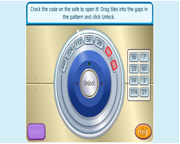Break the Code Algebraic Reasoning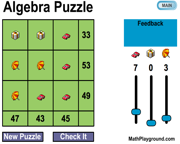Algebra Puzzle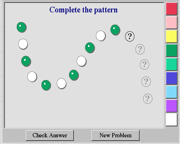Color Pattern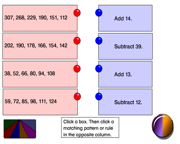Number Patterns and Rules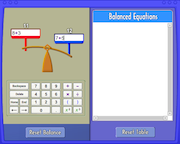Balanced Equations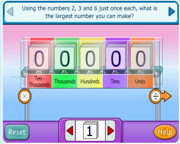Creating Numbers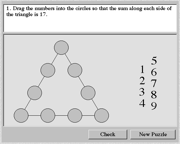Number Puzzles Hoops Thinking Blocks Tutorial Thinking Blocks Tool Grand Slam Math Word Problems Pattern Quest Demolition Division Find the Factorizations
##### Numbers & Operations in Fractions

 Understand equivalent fractions. (4.NF.1) Quarter 1,2,3 and 4 Compare fractions with symbols >,<, or =. (4.NF.2) Quarter 1,2,3 and 4 Add and subtract fractions, including mixed numbers with like denominators. (4.NF.3) Quarter 1,2,3 and 4 Decompose a fraction into a sum of fractions with the same denominator in more than one way. Ex: 3/8=1/8+1/8+1/8; 3/8= 1/8+2/8; 2 1/8= 1+1+1/8= 8/8+8/8+1/8. (4.NF.3) Quarter 1,2,3 and 4 Add and subtract fractions to solve word problems. (4.NF.3) Quarter 1,2,3 and 4 Multiply a fraction by a whole number. Ex: 3 x 2/5= 2/5+2/5+2/5. (4.NF.4) Quarter 1,2,3 and 4 Understand a fraction is a multiple of a unit fraction. Ex: 5/4 as the product of 5 x (1/4). (4.NF.4) Quarter 1,2,3 and 4 Multiply a fraction by a whole number to solve word problems. (4.NF.4) Quarter 1,2,3 and 4 Express and add fractions with denominators 10 and 100. (4.NF.5) Quarter 1,2,3 and 4 Read, write, and represent decimal notation for fractions with denominators 10 or 100. (4.NF.6) Quarter 1,2,3 and 4 Compare two decimals to hundredths using symbols >, <, or = and justify the conclusions. (4.NF.7) Quarter 1,2,3 and 4

Vocabulary

 Denominator: The number below the bar in a fraction that tells how many equal parts are in the whole Compare: Tell how a number is related to another number using <, >, or =. Numerator: The number above the bar in a fraction that tells how many pares of the whole Unit Fraction: one piece of the whole represented as 1/a. Equivalent: (=) having the same value Decimal: A number system (based on 10) with one or more digits to the right of the decimal point. Mixed Number: A number that is made up of a whole number and a fraction Decimal Point: A symbol that seperates dollars and cents in money, and the ones place from the tenths place in decimal numbers.

Activities At Home

• Draw different shapes. Divide them into different fractions.
• Create numbers to use in fractions. Draw these fractions as parts of a whole or set.
• Use measuring cups when baking or cooking.
• Use centimeter paper to draw decimals.
• Relate dimes to tenths and pennies to hundredths and make up decimals using dimes and pennies.
• Use a menu to compare money.
• Roll dice to make decimal numbers and compare them.
• Write decimal numbers in expanded form.
• Write decimal numbers in word form.
• Identify decimals in a newspaper.
• Practice multiplication and division facts.

 Place Value Pirates Comparing Fractions Ordering Decimals Adding Fractions Equivalent Fractions Fraction Race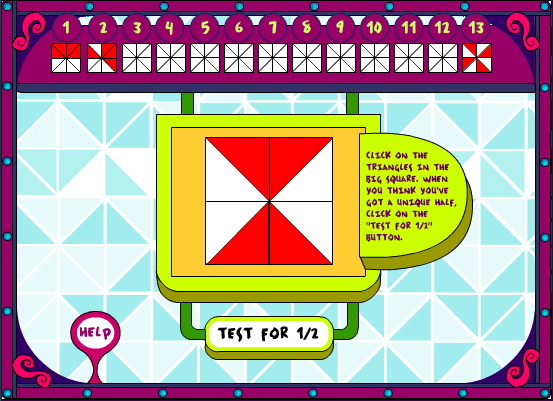Looking at Half Equivalent Fractions Video Equivalent Fractions Video 2 Equivalent Fractions Word Problems Video Adding Fractions Video Adding Fractions Word Problems Unit Fractions Video Multiply Fraction by a Whole Number Multiply a Fraction by a Whole Number Action Fraction Fracton Cafe Triplets Balloon Pop Math Mission Magnetite
##### Geometry

 Identify and draw points, lines, line segments, rays, angles (right, acute, obtuse), and perpendicular and parallel lines in 2-dimensional figures (4.G.1) Quarter 3 and 4 Classify two-dimensional figures. (4.G.2) Quarter 3 and 4 Understand line of symmetry for a two-dimensional figure. (4.G.3) Quarter 3 and 4

Vocabulary

 Point: An exact position or location. Angles: The figure formed when two rays or line segments share the same endpoint. Line: A straight path extending in both directions with no endpoints. Perpendicular Lines: Lines that intersect to form right angles. Line Segments: A part of a line that includes two points, called endpoints, and all the points between them. Parallel Lines: Lines in a plane that never intersect. Ray: A part of a line, with one endpoint, that continues without end in one direction. Two-Dimensional Figure: A closed plane figure with length and width. Symmetry: When one half of a figure looks like the mirror image of the other half.

Activities At Home

• Name two-dimensional figures and find examples at home.
• Identify, describe, and classify different household objects as two-dimensional figures.
• Identify real world angles as acute, right, or obtuse.
• Draw shapes. Identify their angles as obtuse, acute, and right angles.
• Draw pictures with intersecting lines, perpendicular lines, and parallel lines.
• Use tangrams to make two-dimensional figures.
• Practice multiplication and division facts.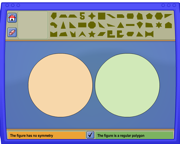Shape Sorter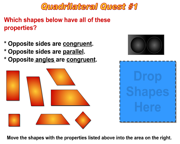Quadrilateral Quest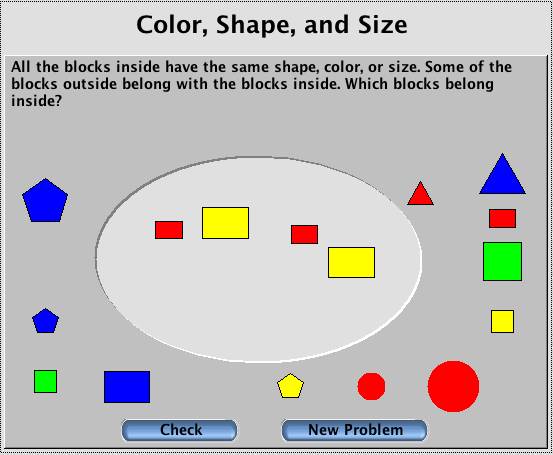Attribute Blocks Geometry Baseball Symmetry Game Symmetry Geometry Memory Toon Angles Angles Shape Sort
##### Measurement

 Convert equivalent measurements in the same measurement system. Ex. 12 in=1 ft. (4.MD.1) Quarter 3 and 4 Solve measurement word problems with distance, time, liquid volumes, masses of objects, and money. (4.MD.2) Quarter 3 and 4 Apply the area and perimeter formulas for rectangles. (4.MD.3) Quarter 3 and 4 Add and subtract fractions on a line plot. (4.MD.4) Quarter 3 and 4 Understand and measure angles. (4.MD.5) Quarter 3 and 4 Measure and draw angles using a protractor. (4.MD.6) Quarter 3 and 4 Break apart an angle into 2 smaller angles and know that the larger angle is the sum of the two smaller angles (4.MD.7) Quarter 3 and 4

Vocabulary

 Perimeter: The distance around and object. Capacity: The greatest amount a container can hold. Area: The measure, in square units, of the inside of a plane figure. Line Plot: A graph that shows the frequency of data along a number line. Volume: The number of cubic units that fits inside a solid figure. Angles: The figure formed when two rays or line segments share the same endpoint. Elapsed Time: The amount of time that passes between two times. Protractor: A tool used to measure angles.

Activities At Home

• Use measurement tools when baking or cooking.
• Compare items by length or weight.
• Practice scheduling events to determine elapsed time.
• Use string to measure wrist, neck, and waist and make comparisons.
• Read an analog clock throughout the day.
• Use a stopwatch to keep track on how much T.V is watched throughout the week and how much .time is spent on homework, and compare the two amounts of time.
• Take an object and estimate the weight then use a scale to determine the exact weight, and compare the two amounts.
• Use a ruler to measure objects around the house in inches or centimeters.
• Explore the area and volume of a cereal box.
• Identify real world angles as acute, right, or obtuse.
• Draw shapes. Identify their angles as obtuse, acute, and right angles.
• Draw pictures with intersecting lines, perpendicular lines, and parallel lines.
• Use a ruler to find a perimeter of plane figures.
• Practice multiplication and division facts.

 Perimeter and Area Shodor Area and Perimeter Multiple Measures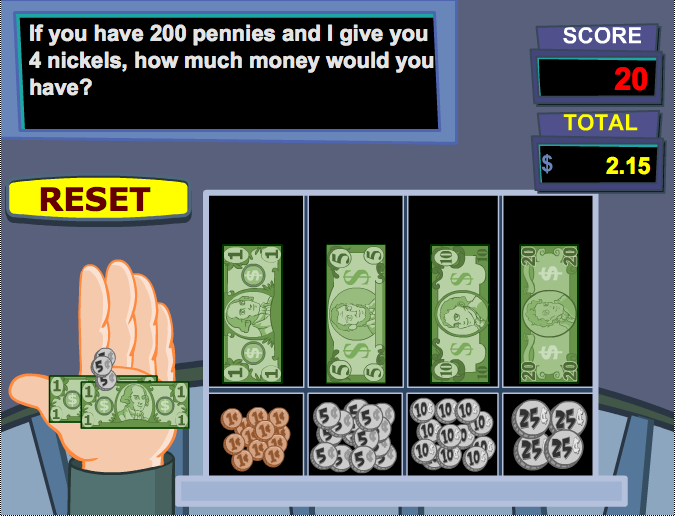Adding Money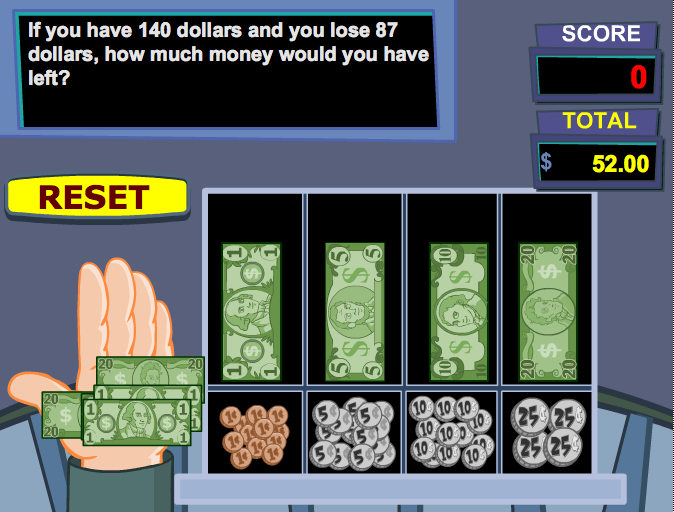Subtracting Money Area and Perimeter Angles Ruler Game Attack Angle Geometry Baseball